GATE  >  Test: Combinational Logic Circuits

# Test: Combinational Logic Circuits

Test Description

## 10 Questions MCQ Test Digital Circuits | Test: Combinational Logic Circuits

Test: Combinational Logic Circuits for GATE 2023 is part of Digital Circuits preparation. The Test: Combinational Logic Circuits questions and answers have been prepared according to the GATE exam syllabus.The Test: Combinational Logic Circuits MCQs are made for GATE 2023 Exam. Find important definitions, questions, notes, meanings, examples, exercises, MCQs and online tests for Test: Combinational Logic Circuits below.
Solutions of Test: Combinational Logic Circuits questions in English are available as part of our Digital Circuits for GATE & Test: Combinational Logic Circuits solutions in Hindi for Digital Circuits course. Download more important topics, notes, lectures and mock test series for GATE Exam by signing up for free. Attempt Test: Combinational Logic Circuits | 10 questions in 30 minutes | Mock test for GATE preparation | Free important questions MCQ to study Digital Circuits for GATE Exam | Download free PDF with solutions
 1 Crore+ students have signed up on EduRev. Have you?
Test: Combinational Logic Circuits - Question 1

### Which device converts, a decimal input number to binary

Detailed Solution for Test: Combinational Logic Circuits - Question 1

An encoder converts information from one format or code to another. An encoder can be used to encode the decimal number to binary number.

Test: Combinational Logic Circuits - Question 2

### A multiplexer is a

Detailed Solution for Test: Combinational Logic Circuits - Question 2

Combinational Logic circuits are circuits for which the present output depends only on the present input, i.e. there is no memory element to store the past output.

A combinational circuit can have ‘n’ number of inputs and ‘m’ number of outputs as shown: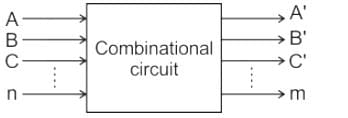Combinational circuits are:

• Multiplexer/Demultiplexer
• Encoder/Decoder
• Subtractors
• Code Converters

Multiplexers:

• A multiplexer is Many to one data selector.
• A multiplexer selects one of the many data available at its input depending on the bits on the select line.
• For 2m inputs, there are m select lines that determine which input is to be connected to the output.
Test: Combinational Logic Circuits - Question 3

### 32 decoder circuit can be implemented with ______.

Detailed Solution for Test: Combinational Logic Circuits - Question 3

Concept:

• A decoder is a combinational logic constructed with logic gates.
• It is the reverse of the encoder.
• A decoder circuit is used to transform a set of digital input signals into an equivalent decimal code of its output. For ‘n’ inputs a decoder gives ‘2n’ outputs.
• Block diagram of the Decoder is shown below: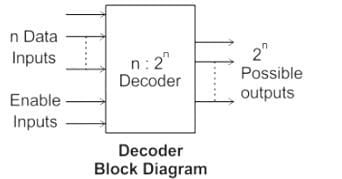Decoder expansion

n1 × m1 → n2 × m2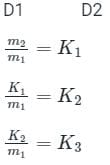The number of D2 decoder required is given as:

∑ K = K1 + K2 + K3 + ------

Calculation:

One 2:4 decoder and four 3:8 decoders can represent 5:32 decoder in the following way: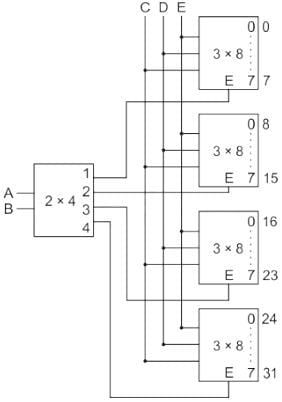Hence option (A) is the correct answer.

Test: Combinational Logic Circuits - Question 4

Which of the following circuit has its output dependent only upon the present input?

Detailed Solution for Test: Combinational Logic Circuits - Question 4

Combinational Logic circuits are circuits for which the present output depends only on the present input, i.e. there is no memory element to store the past output.

A combinational circuit can have ‘n’ number of inputs and ‘m’ number of outputs as shown:Combinational circuits are:

• Multiplexer/Demultiplexer
• Encoder/Decoder
• Subtractors
• Code Converters

In a sequential circuit, the output depends on both the present and the past values. The circuit diagram is as shown: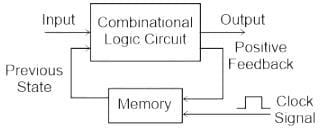Examples of sequential circuits:

• Shift Registers
• Flip flops
• Counters
Test: Combinational Logic Circuits - Question 5

Construct a 16 × 1 multiplexer using 2 × 1 multiplexer. How many 2 × 1 multiplexer are required to construct?

Detailed Solution for Test: Combinational Logic Circuits - Question 5

To implement 2n × 1 MUX using 2 × 1 MUX, the total number of 2 × 1 MUX required is (2n - 1).

∴ The number of 2 × 1 multiplexer required to implement 16 × 1 MUX will be:

n = 16 - 1 = 15

Or we can follow the below steps to calculate the same:

1st stage = 16/2 = 8

2nd  stage = 8/2 = 4

3rd stage = 4/2 = 2

4th stage = 2/2 = 1

The sum will give the total number of MUX required to implement 16 × 1 multiplexer using 2 × 1, i.e.

n = 8 + 4 + 2 + 1 = 15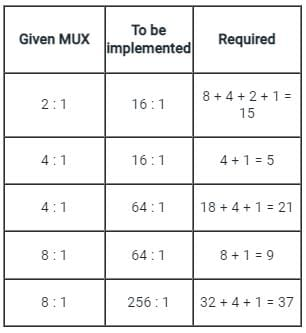Test: Combinational Logic Circuits - Question 6

A combinational circuit is the one in which output depends on the

Detailed Solution for Test: Combinational Logic Circuits - Question 6

Combinational Logic circuits:

The Combinational Logic circuits are circuits for which the present output depends only on the present input.

There is no memory element to store the past output.

It performs an operation that can be specified logically by a set of Boolean functions.

A combinational circuit can have ‘n’ number of inputs and 'm' number of outputs as shown:Hence, All the above statements are correct.

Sequential Circuits: The block diagram shown below explains this:The memory elements used are flip flops or latches.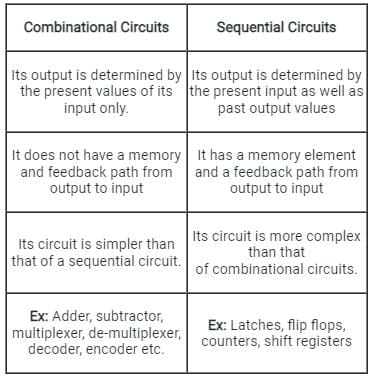Test: Combinational Logic Circuits - Question 7

AND gate EXOR gate combination is ____

Detailed Solution for Test: Combinational Logic Circuits - Question 7

A half adder circuit is basically made up of and a AND gate with an XOR gate as shown below.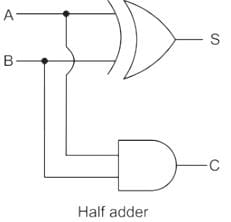• A half adder is also known as XOR gate because XOR is applied to both inputs to produce the sum
• Half adder can add only two bits (A and B) and has nothing to do with the carry
• If the input to a half adder has a carry, then it will neglect it and adds only the A and B bits
• That means the binary addition process is not complete and that's why it is called a half adder

Sum (S) = A⊕B, Carry = A.B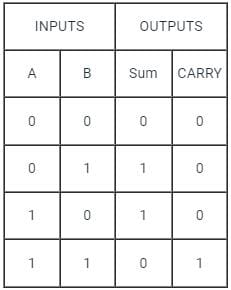Test: Combinational Logic Circuits - Question 8

In a half adder, the carry output is high if the inputs are:

Detailed Solution for Test: Combinational Logic Circuits - Question 8

The truth table for half adder is given below: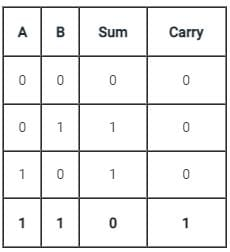Therefore, the carry is given by AB.

Hence the carry output is high if the both inputs are high i.e. 1,1

Test: Combinational Logic Circuits - Question 9

In half adder, the total number of inputs and outputs are:

Detailed Solution for Test: Combinational Logic Circuits - Question 9

Concept:

A half adder circuit is basically made up of and a AND gate with XOR gate as shown below.• A half adder is also known as the XOR gate because XOR is applied to both inputs to produce the sum.
• Half adder can add only two bits (A and B) and has nothing to do with the carry.
• Half adder has two inputs (A and B) and two outputs (S and C).
• If the input to a half adder has a carry, then it will neglect it and adds only the A and B bits.
• That means the binary addition process is not complete and that's why it is called a half adder.
Test: Combinational Logic Circuits - Question 10

For the multiplexer shown below, the number of selection lines is equal to ________?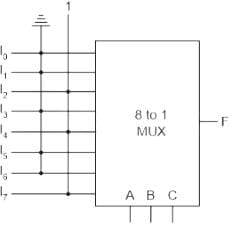Detailed Solution for Test: Combinational Logic Circuits - Question 10

Concept:

Multiplexer:

• It is type of combinational circuit for which the present output depends only on the present input.
• It is also known as memoryless circuit because there are no memory element to store the past output.
• A multiplexer is a many to one data selector.
• It selects one of the many data available at its input depending on the bits of the select lines.
• For 2n inputs, there are n select lines that determine which input is to be connected to the output.In the given multiplexer circuit, we have 8 input lines.

∴ 2n = 8

2= 23

By comparing exponents on both sides we get;

n = 3

In conclusion, the total number of select lines is (n) = 3

## Digital Circuits

76 docs|43 tests
 Use Code STAYHOME200 and get INR 200 additional OFF Use Coupon Code
Information about Test: Combinational Logic Circuits Page
In this test you can find the Exam questions for Test: Combinational Logic Circuits solved & explained in the simplest way possible. Besides giving Questions and answers for Test: Combinational Logic Circuits, EduRev gives you an ample number of Online tests for practice

76 docs|43 tests